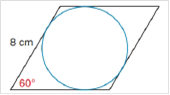Chapter 8.4, Problem 47EElementary Geometry For College St...

7th Edition
Alexander + 2 others
ISBN: 9781337614085

Solutions

Chapter
SectionElementary Geometry For College St...

7th Edition
Alexander + 2 others
ISBN: 9781337614085
Textbook Problem

Given that the length of each side of a rhombus is 8 cm and that an interior angle measures 60°. Find the exact area of the inscribed circle.To determine

To Find:

The area of the circle inscribed in a rhombus.

Explanation

The side length of the rhombus is 8 cm.

The angle of the rhombus is 60°.

The diagonals of the rhombus AC and BD bisect each other with right angles at the center of the circle.

The diagonals divide the rhombus in to four 30-60-90 triangles.

In the triangle AOB, We know AB = 8.

In 30-60-90 triangles, the side length ratio is 1:2:3.

Therefore, the length of OB = 4 and OA is 43.

Now, draw a perpendicular OP (radius of the circle) to the side AB of rhombus.

It forms a new 30-60-90 right angle triangle AOP.

Now, we know one side OA is 43

Still sussing out bartleby?

Check out a sample textbook solution.

See a sample solution

The Solution to Your Study Problems

Bartleby provides explanations to thousands of textbook problems written by our experts, many with advanced degrees!

Get Started

Evaluate the integrals in Problems 1-26.

Mathematical Applications for the Management, Life, and Social Sciences